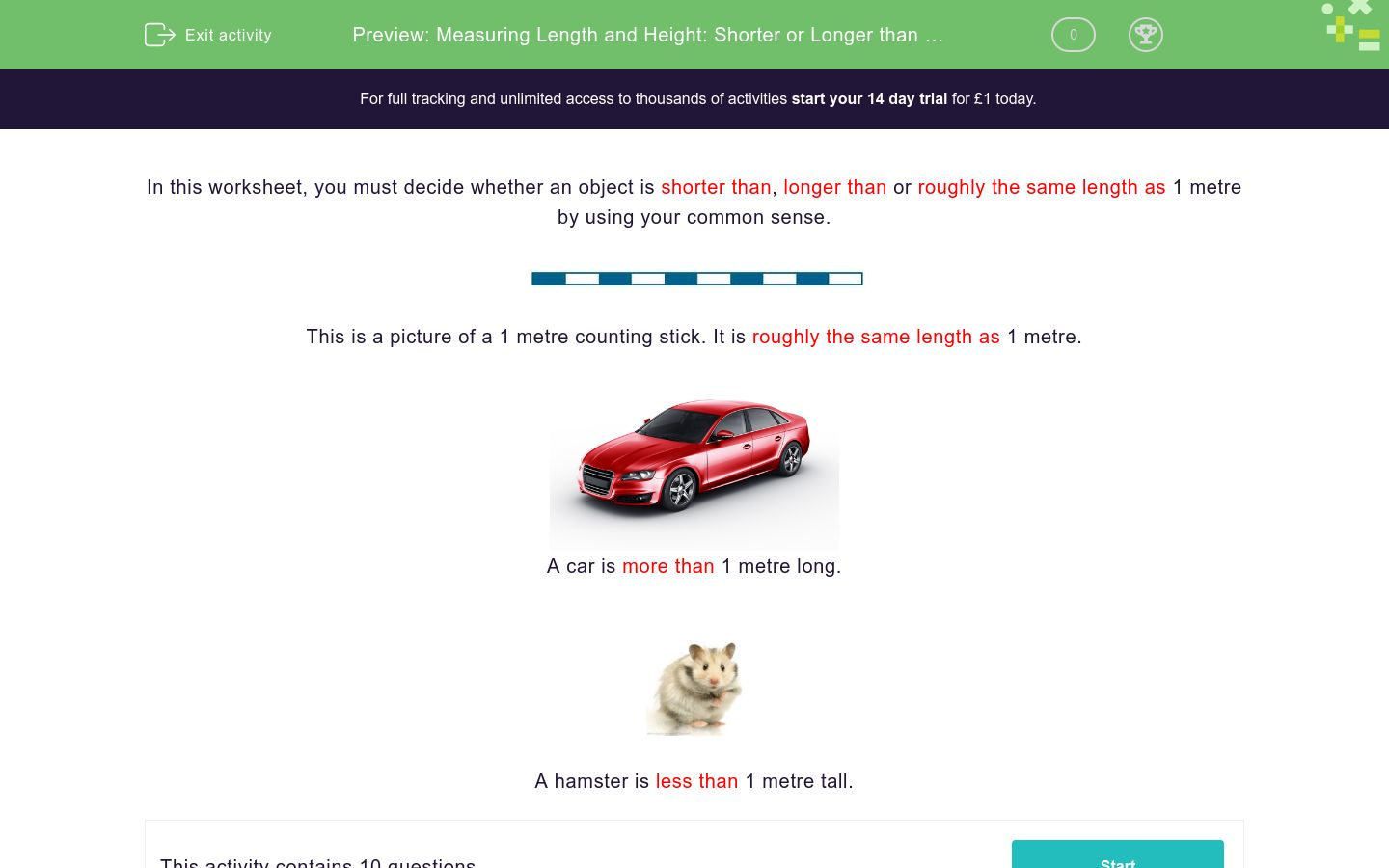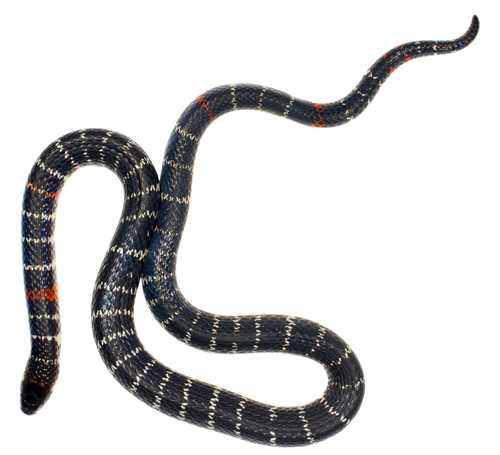# Measuring Length and Height: Shorter or Longer than 1 Metre?

In this worksheet, students decide whether an object is shorter than, longer than or roughly the same length as 1 metre.Key stage:  KS 1

Curriculum topic:   Measurement

Curriculum subtopic:   Solve Measurement Problems

Difficulty level:### QUESTION 1 of 10

In this worksheet, you must decide whether an object is shorter thanlonger than or roughly the same length as 1 metre by using your common sense.This is a picture of a 1 metre counting stick. It is roughly the same length as 1 metre.A car is more than 1 metre long.A hamster is less than 1 metre tall.

Is this object shorter thanlonger than or roughly the same length as a 1 metre stick?A banana

Shorter than 1m

Longer than 1m

Roughly the same as 1m

Is this object shorter thanlonger than or roughly the same length as a 1 metre stick?A duck

Shorter than 1m

Longer than 1m

Roughly the same as 1m

Is this object shorter thanlonger than or roughly the same length as a 1 metre stick?A giant snake

Shorter than 1m

Longer than 1m

Roughly the same as 1m

Is this object shorter thanlonger than or roughly the same length as a 1 metre stick?A matchstick

Shorter than 1m

Longer than 1m

Roughly the same as 1m

Is this object shorter thanlonger than or roughly the same length as a 1 metre stick?A 1 metre tape measure

Shorter than 1m

Longer than 1m

Roughly the same as 1m

Is this object shorter thanlonger than or roughly the same length as a 1 metre stick?A tea cup

Shorter than 1m

Longer than 1m

Roughly the same as 1m

Is this object shorter thanlonger than or roughly the same length as a 1 metre stick?A bunch of grapes

Shorter than 1m

Longer than 1m

Roughly the same as 1m

Is this object shorter thanlonger than or roughly the same length as a 1 metre stick?A bed

Shorter than 1m

Longer than 1m

Roughly the same as 1m

Is this object shorter thanlonger than or roughly the same length as a 1 metre stick?A caterpillar

Shorter than 1m

Longer than 1m

Roughly the same as 1m

Is this object shorter thanlonger than or roughly the same length as a 1 metre stick?A camel

Shorter than 1m

Longer than 1m

Roughly the same as 1m

• Question 1

Is this object shorter thanlonger than or roughly the same length as a 1 metre stick?A banana

Shorter than 1m
• Question 2

Is this object shorter thanlonger than or roughly the same length as a 1 metre stick?A duck

Shorter than 1m
• Question 3

Is this object shorter thanlonger than or roughly the same length as a 1 metre stick?A giant snake

Longer than 1m
• Question 4

Is this object shorter thanlonger than or roughly the same length as a 1 metre stick?A matchstick

Shorter than 1m
• Question 5

Is this object shorter thanlonger than or roughly the same length as a 1 metre stick?A 1 metre tape measure

Roughly the same as 1m
• Question 6

Is this object shorter thanlonger than or roughly the same length as a 1 metre stick?A tea cup

Shorter than 1m
• Question 7

Is this object shorter thanlonger than or roughly the same length as a 1 metre stick?A bunch of grapes

Shorter than 1m
• Question 8

Is this object shorter thanlonger than or roughly the same length as a 1 metre stick?A bed

Longer than 1m
• Question 9

Is this object shorter thanlonger than or roughly the same length as a 1 metre stick?A caterpillar

Shorter than 1m
• Question 10

Is this object shorter thanlonger than or roughly the same length as a 1 metre stick?A camel

Longer than 1m
---- OR ----

Sign up for a £1 trial so you can track and measure your child's progress on this activity.

### What is EdPlace?

We're your National Curriculum aligned online education content provider helping each child succeed in English, maths and science from year 1 to GCSE. With an EdPlace account you’ll be able to track and measure progress, helping each child achieve their best. We build confidence and attainment by personalising each child’s learning at a level that suits them.

Get started